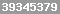Share

# Microsoft Excel opens new worksheets in the same window

 Microsoft Excel displays new worksheet tabs in the same window - resulting in many tabs in one open window if more than one file is open. Problem: Microsoft Excel opens each new worksheet in the same window.MIcrosoft Excel I want each new file to open in a new Excel program window, so that each new worksheet excel does not open in the same window. Solution: To fix this, you need to tell Excel that you want each new window to open separately from your old file. Options >> View >> CHECK "Windows in Task bar"

 Home  |  Search  |  ContactShare  |  Help  |  Sitemap## Best of Site Popular Calculators

Find Percentage with Percent Increase Online Calculator: Calculate Percent Increase - our most popular online calculator

Calculate percent of two numbers: percent of number using simple ratio: Calculate Percent % of Many Numbers

Percent Calculator and Percentage Formula to calculate percent of a number: Calculate Percent % of a Number

Calculate Percent of Total: Find percentage of total

## Presidents on Bills and Coins

Presidents on money

Presidents on coins

Most expensive paper money: Grand Watermelon